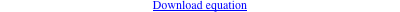too complicated to be written here. Click on the link to download a text file.X(2), X(3), X(20), X(6200), X(6396), X(13712), X(13835), X(39162), X(39163), X(39164), X(39165), X(55157), X(55158), X(55159) foci of the Steiner inellipse vertices of the Thomson triangle antipodes of points of K006 on (O) infinite points of pK(X6, X11001) vertices of the outer and inner Vecten triangles Geometric properties :See Table 81 for general properties. K1331 is the cubic F(T) obtained when T = 2. K1331 is the Thomson-isogonal transform of K1329.